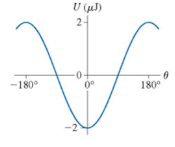# Problem: The graph shows the potential energy of an electric dipole. Consider a dipole that oscillates between ±60°What is dipole's kinetic energy when it is aligned with the electric field if the mechanical energy is -1μJ and potential energy of the system is 2μJ.

###### FREE Expert Solution

Potential energy:

$\overline{){{\mathbf{U}}}_{\mathbf{d}\mathbf{i}\mathbf{p}\mathbf{o}\mathbf{l}\mathbf{e}}{\mathbf{=}}{\mathbf{-}}{\mathbf{P}}{\mathbf{E}}{\mathbf{c}}{\mathbf{o}}{\mathbf{s}}{\mathbf{\theta }}}$

Total energy:

$\overline{){\mathbf{E}}{\mathbf{=}}{\mathbf{K}}{\mathbf{E}}{\mathbf{+}}{\mathbf{U}}}$

The dipole is aligned with the electric field when θ = 0°.

92% (87 ratings)###### Problem Details

The graph shows the potential energy of an electric dipole. Consider a dipole that oscillates between ±60°What is dipole's kinetic energy when it is aligned with the electric field if the mechanical energy is -1μJ and potential energy of the system is 2μJ.

Frequently Asked Questions

What scientific concept do you need to know in order to solve this problem?

Our tutors have indicated that to solve this problem you will need to apply the Dipole Moment concept. You can view video lessons to learn Dipole Moment. Or if you need more Dipole Moment practice, you can also practice Dipole Moment practice problems.

What professor is this problem relevant for?

Based on our data, we think this problem is relevant for Professor Sotero's class at UCF.